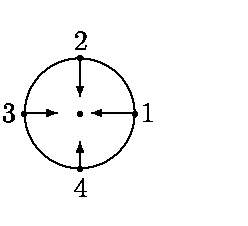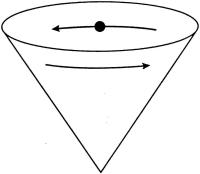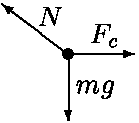# Centripetal Force Theory Questions

• DanielleG

## Homework Statement

1. Answer True, False, or Cannot tell to each of the five statements below.
1. A small projectile is launched horizontally 1 m above the surface of a smooth, airless planet, with sufficient speed for orbit. A bug riding in a small hole in the projectile has apparent weight equal to zero.
2. A ball on a string moves in a vertical circle. When the ball is at its highest point, the tension in the string is less than the ball's weight.
3. The figure shows the radial acceleration ar at four sequential points on the trajectory of a particle moving in a circle. The tangential acceleration at point 3 points up.4. All points on a rotating wheel have the same radial acceleration.
5. A marble rolls around the inside of a cone. For the marble at the right side of the cone, the free body diagram is correct.## Homework Equations

Not many equations for this, just theory

## The Attempt at a Solution

1) True! Apparent weight = 0 N in free fall.
2) Unsure, but am leaning towards false.
3) Cannot tell! Depends on which way the particle is moving.
4) False, depends on the way the wheel is rotating.
5) False, Fc should be pointing to the left, not the right.

I have only one try left, and I'm just very unsure about b on the most part. Any guidance concerning #2? Thanks in advance!

For number two think what is actually happening when the ball is at the highest point. Draw a force diagram for the ball at the highest point and note what force is holding the ball down and what is holding it up (tension).

For 3 and 4 you have the right answer but the reason you offer is wrong.
For 5, is it clear what the c in Fc stands for?

Draw a force diagram for the ball at the highest point and note what force is holding the ball down and what is holding it up (tension).

I've drawn a diagram and I know that at the top both the weight and tension are pointing down, but I am unsure of how to know which is smaller or larger.

For 3 and 4 you have the right answer but the reason you offer is wrong.

Hmmmm, okay, I'll give it some thought.

For 5, is it clear what the c in Fc stands for?

I am basically positive it stands for centripetal force.

I've drawn a diagram and I know that at the top both the weight and tension are pointing down,

Correct!

The sum of those two forces equals ...

The sum of those two forces equals ...

The centripetal force! So Fc = Ft + Fg, which can be rearranged to give Ft = Fc - Fg. Does that mean the tension in the string is greater than the ball's weight?

The centripetal force! So Fc = Ft + Fg,

Correct!

which can be rearranged to give Ft = Fc - Fg.

I don't see how that rearrangement helps determine if Ft is less than Fg.

Does that mean the tension in the string is greater than the ball's weight?

Think about the ball's speed if Ft = 0. This is the minimum speed needed to maintain circular motion, a question sometimes asked on tests!

Last edited:
Think about the ball's speed if Ft = 0. This is the minimum speed needed to maintain circular motion, a question asked on test questions!

So the minimum speed needed to keep a ball in circular motion occurs when Ft = 0. Does that mean the weight is what's keeping the ball going in a circular motion? And if that's the case, then the tension in the string is definitely less than the ball's weight.

So the minimum speed needed to keep a ball in circular motion occurs when Ft = 0. Does that mean the weight is what's keeping the ball going in a circular motion?

When the ball is at that position, yes.

And if that's the case, then the tension in the string is definitely less than the ball's weight.

And if the speed is larger than that minimum value Ft will be larger. Anything happen if Ft is larger yet and equal to Fg? Larger than that? What can you say about the ball's speed in each of these cases?

Last edited:
Anything happen if Ft is larger yet and equal to Fg? Larger than that? What can you say about the ball's speed in each of these cases?

Hmmm, would v get larger as Ft becomes larger?

Hmmm, would v get larger as Ft becomes larger?

You got it!

The problem statement
The figure shows the radial acceleration ar at four sequential points on the trajectory of a particle moving in a circle. The tangential acceleration at point 3 points up.The attempt at a solution

Cannot tell! Depends on which way the particle is moving.

Suppose the particle is moving in the clockwise direction. If the tangential component of the acceleration points upward the particle is speeding up. If downward the particle is slowing down.

If the particle is moving in the counter-clockwise direction we would have the opposite situation.

In general, if the directions of the velocity and the tangential component of the acceleration are the same, the particle is speeding up.

If the directions of the velocity and the tangential component of the acceleration are opposite, the particle is slowing down.

The problem statement
All points on a rotating wheel have the same radial acceleration.

The attempt at a solution
False, depends on the way the wheel is rotating.

Points on the wheel that are different distances from the center will have different radial accelerations.

a
c = v2/r.

You got it!
Thank you!